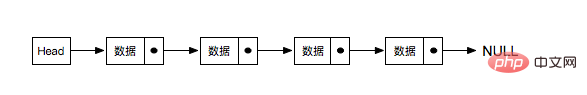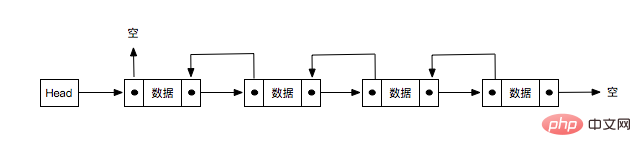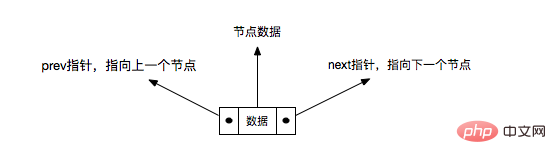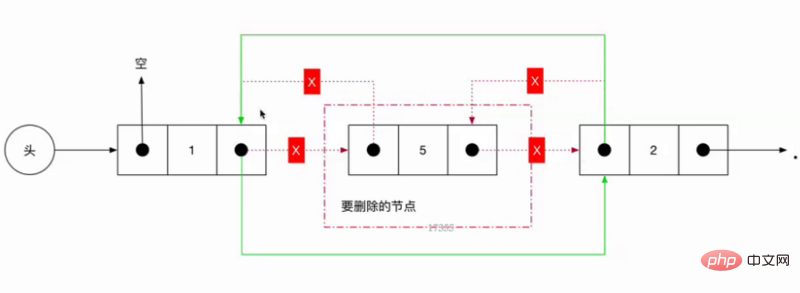• 首页
• 更多

# JavaScript中链表的详细介绍

```    insert(arr, index, data){
for(let i = index + 1; i < arr.length; i++){
arr[i] = arr[i - 1];
}
arr[index] = data;
}```

### 单向链表#### 单向链表的特点：

• 用一组任意的内存空间去存储数据元素（这里的内存空间可以是连续的，也可以是不连续的）

• 每个节点(node)都由数据本身和一个指向后续节点的指针组成

• 整个链表的存取必须从头指针开始，头指针指向第一个节点

• 最后一个节点的指针指向空（NULL）

• 创建节点

• 插入节点

• 搜索/遍历节点

• 删除节点

• 合并

#### 初始化节点

• 指针指向空

• 存储数据

```    class Node {
constructor(key) {
this.next = null;
this.key = key;
}
}```

#### 初始化单向链表

• 每个链表都有一个头指针，指向第一个节点，没节点则指向NULL

```    class List {
constructor() {
this.head = null;
}
}```

#### 创建节点

```    static createNode(key) {
return new createNode(key);
}```

#### 插入节点（插入到头节点之后）

• head没有指向任何节点，说明当前插入的节点是第一个

• head指向新节点

• 新节点的指针指向NULL

• head有指向的节点

• head指向新的节点

• 新节点的指针指向原本head所指向的节点

```    insert(node) {
// 如果head有指向的节点
if(this.head){
node.next = this.head;
}else {
node.next = null;
}
this.head = node;
}```

### 搜索节点

• 从head开始查找

• 找到节点中的key等于想要查找的key的时候，返回该节点

```    find(key) {
let node = this.head;
while(node !== null && node.key !== key){
node = node.next;
}
return node;
}```

### 删除节点

• 所要删除的节点刚好是第一个，也就是head指向的节点

• 将head指向所要删除节点的下一个节点(node.next)

• 要删除的节点为最后一个节点

• 寻找到所要删除节点的上一个节点(prevNode)

• 将prevNode中的指针指向NULL

• 在列表中间删除某个节点

• 寻找到所要删除节点的上一个节点(prevNode)

• 将prevNode中的指针指向当前要删除的这个节点的下一个节点

```    delete(node) {
// 第一种情况
if(node === this.head){
this.head = node.next;
return;
}

// 查找所要删除节点的上一个节点
let prevNode = this.head;
while (prevNode.next !== node) {
prevNode = prevNode.next;
}

// 第二种情况
if(node.next === null) {
prevNode.next = null;
}

// 第三种情况
if(node.next) {
prevNode.next = node.next;
}
}```

### 单向链表整体的代码

```class ListNode {
constructor(key) {
this.next = null;
this.key = key;
}
}

class List {
constructor() {
this.head = null;
this.length = 0;
}

static createNode(key) {
return new ListNode(key);
}

// 往头部插入数据
insert(node) {
// 如果head后面有指向的节点
if (this.head) {
node.next = this.head;
} else {
node.next = null;
}
this.head = node;
this.length++;
}

find(key) {
let node = this.head;
while (node !== null && node.key !== key) {
node = node.next;
}
return node;
}

delete(node) {
if (this.length === 0) {
throw 'node is undefined';
}

if (node === this.head) {
this.head = node.next;
this.length--;
return;
}

let prevNode = this.head;

while (prevNode.next !== node) {
prevNode = prevNode.next;
}

if (node.next === null) {
prevNode.next = null;
}
if (node.next) {
prevNode.next = node.next;
}
this.length--;
}
}```

### 双向链表#### 初始化节点

• 指向前一个节点的指针

• 指向后一个节点的指针

• 节点数据

```    class ListNode {
this.prev = null;
this.next = null;
this.key = key;
}```

#### 初始化双向链表

• 头指针指向NULL

```    class List {
constructor(){
this.head = null;
}
}```

#### 创建节点

```    static createNode(key){
return new ListNode(key);
}```

#### 插入节点（（插入到头节点之后）

• 看上图中head后面的第一个节点可以知道，该节点的prev指向NULL

• 节点的next指针指向后一个节点, 也就是当前头指针所指向的那个节点

• 如果head后有节点，那么原本head后的节点的prev指向新插入的这个节点(因为是双向的嘛)

• 最后将head指向新的节点

```    insert(node) {
node.prev = null;
node.next = this.head;
if(this.head){
this.head.prev = node;
}
this.head = node;
}```

#### 搜索节点

```  search(key) {
let node = this.head;
while (node !== null && node.key !== key) {
node = node.next;
}
return node;
}```

#### 删除节点

• 删除的是第一个节点

• head指向所要删除节点的下一个节点

• 下一个节点的prev指针指向所要删除节点的上一个节点

• 删除的是中间的某个节点

• 所要删除的前一个节点的next指向所要删除的下一个节点

• 所要删除的下一个节点的prev指向所要删除的前一个节点

• 删除的是最后一个节点

• 要删除的节点的上一个节点的next指向null（也就是指向删除节点的next所指的地址）```    delete(node) {
const {prev,next} = node;
delete node.prev;
delete node.next;
if(node === this.head){
this.head = next;
}
if(next){
next.prev = prev;
}
if(prev){
prev.next = next;
}
}```

### 双向链表整体代码

```    class ListNode {
constructor(key) {
// 指向前一个节点
this.prev = null;
// 指向后一个节点
this.next = null;
// 节点的数据(或者用于查找的键)
this.key = key;
}
}

/**
* 双向链表
*/
class List {
constructor() {
this.head = null;
}

static createNode(key) {
return new ListNode(key);
}

insert(node) {
node.prev = null;
node.next = this.head;
if (this.head) {
this.head.prev = node;
}
this.head = node;
}

search(key) {
let node = this.head;
while (node !== null && node.key !== key) {
node = node.next;
}
return node;
}

delete(node) {
const { prev, next } = node;
delete node.prev;
delete node.next;

if (node === this.head) {
this.head = next;
}

if (prev) {
prev.next = next;
}
if (next) {
next.prev = prev;
}
}
}```

### 总结

• Python中hasattr(),getattr(),setattr()的用法介绍（代码示例）
• python装饰器是什么？怎么用？
• 2018年度最热门的编程工具下载资源汇总
• php中array_map()函数如何使用？（代码示例）
• Python处理gif动态图的解析与合成操作的介绍
• canvas中普通动效与粒子动效的实现 方法介绍（代码示例）
• H5使用react组件实现拍照、选择图片上传
• RxJS实现Redux Form的详细介绍（代码示例）
• 最新文章